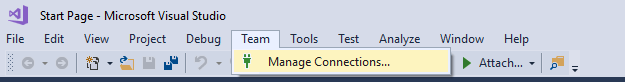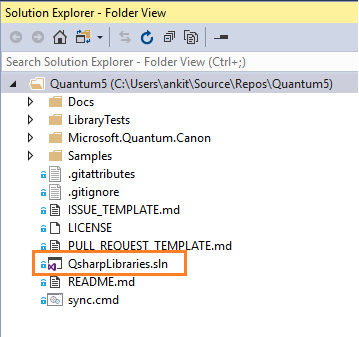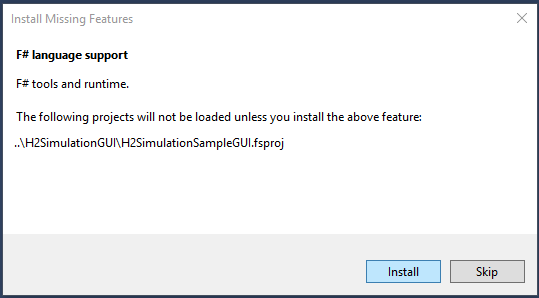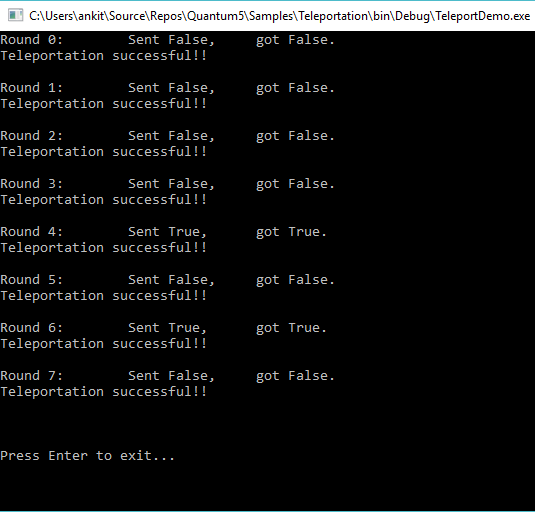# An Introduction to Q#

### Q# is an accessible language for quantum computing--cool, right? Come join us as we take an in-depth look at this language.

· Integration Zone · Presentation
Save
9.74K Views

### Introduction

In this article, I will be talking about Q# – the new programming language introduced by Microsoft for quantum computing. We will go over the data types, expressions, and statements of Q# with the help of code snippets.

### Prerequisites

Please visit my earlier article An Introduction To Quantum Computing to get a basic understanding of Quantum Computing and to also know how to install Quantum Development Kit in Visual Studio 2017.

### What Is Q#?

According to Microsoft, Q# is a scalable, multi-paradigm, domain-specific programming language for quantum computing.

So, what do these terms actually mean? Let us dive into the details.

• ScalableQ# allows us to write code that can be executed on machines of varying computing abilities. We can use it to simulate a few Qubits on our local machine or even thousands of Qubits for an enterprise level application.
• Multi-paradigmQ# is a multi-paradigm programming language as it supports functional as well as imperative programming styles. If you are new to this programming paradigm, refer to the Wikipedia article here for a good introduction.
• Domain-specificQ# is a programming language for quantum computing. It is to be used for writing algorithms/code snippets to be executed on quantum processors.

### Validating a Q# Development Environment on Your Machine

As this point in time, I assume that you have already installed the Quantum Development Kit (QDK). If not, then please do it by referring to the prerequisites section.

After you have successfully installed QDK, we need to verify if VS 2017 has all the required dependencies installed to start with Q# development. For this, we will clone and execute the quantum sample programs from GitHub provided by Microsoft.

Open VS 2017 and navigate to Team >> Manage Connections.Select Clone under Local Git Repositories and enter the URL,  https://github.com/Microsoft/Quantum.git, and click “Clone.”The repository will be cloned on your local computer and Visual Studio will switch to the Solution Explorer displaying all the cloned libraries and samples.Now, open the QsharpLibraries.sln solution. If you are prompted with the “Install Missing Features popup box, click “Install” to allow the installation of the necessary features. This will download and install F# and other tools used by some of the samples. Make sure that you are connected to the internet.To execute a sample program, right-click on the TeleportationSample project in “Samples > 0.Introduction folder” of QsharpLibraries solution, and then click on “Set as Startup Project” and press F5.If you can see an output screen similar to the one shown below, then, congratulations, your VS 2017 is ready for Q# development!Note that your output screen may vary because the data that is being teleported is random. But it should successfully send 8 rounds of data.

### Q# Type Model

Let us go over the various type models provided by Q#.

#### Primitive Types

• Int: It represents the 64-bit signed integer. Notice the upper case ‘I’. This is in contrast to int in C# with a lower case ‘i’.
• Double: It represents double-precision floating point number. This also has an upper case ‘D’ in contrast to double in C#.
• Bool: It represents the Boolean type and can take two values – true or false.
• Qubit: This represents the Quantum bit. Qubit is the fundamental unit of processing information in quantum computers, similar to a bit in classical computers.
• Pauli: This type is used to denote the base operation for rotations and to specify the basis of a measurement. This can take four possible values: PauliI, PauliX, PauliY, PauliZ.
• Result: This represents the result of a measurement. This can take two possible values, Zero or One.
• Range: This represents a sequence of integers.
• String: It represents a sequence of Unicode characters.

#### Array Type

We can create an array type of any valid Q# primitive type. Q# does not support rectangular multi-dimensional arrays, instead, it supports only jagged arrays.

e.g.: Int[], Qubit[][]

By default, all variables in Q# are immutable, i.e. their values cannot be changed after they are bound. Hence, to create an array whose values can be set, we will use the `mutable` keyword

e.g. `mutable myArr=new Int ;`

This will create an integer array, `myArr`, of size 5. The elements of a new array are initialized to a type-dependent default value, in this case, it will be 0, the default value for an integer type.

Arrays passed as arguments are immutable. All arrays in Q# are zero-based, i.e., the first element of an array, `arr`, is always `arr`.

#### Tuple Type

Tuple type represents a tuple of values of any given primitive type. It is represented as (T1, T2, T3,…) where T1, T2, T3 is a primitive type. The Q# tuple is immutable, i.e. we cannot change the contents of the tuple once it has been created.

A tuple expression can contain values of multiple primitive types, i.e. a tuple of type (Int, Double, Result) is a valid tuple.

We can create a tuple with a single element also, like (2). This is known as a singleton tuple and it is considered equal to the value of an enclosed type. This property is called 'singleton tuple equivalence.'

e.g., (2) is a singleton tuple of type Int, but it is considered equivalent to an integer 2.

#### User Defined Type

We can create a user-defined type of any primitive type. We can also create an array of user-defined types or we can include it in a tuple. User-defined types cannot have a cyclic dependency on each other, i.e. it is not possible to create a recursive type structure, since a user-defined type is a subtype of the base type. Hence, it can be used anywhere a value of the base type is expected.

#### Operation Type

A Q# operation is a callable routine, which contains Q# code to carry out a quantum operation. An operation is the basic unit of quantum execution in Q#. The operation can only take a single value as input in the form of a tuple and return a single value as output specified after a colon and may be a tuple.

An operation has a body section, which contains the implementation of the operation. It can also have adjoint, controlled, and controlled adjoint sections. These are used to specify specific variants of appropriate operations. The arguments to an operation are specified as a tuple, within parentheses. The return type of the operation is as shown in the sample operation below:

``````operation AddInteger(a: Int, b: Int): Int {
body {
mutable c = 0;
set c = a + b;
return (c);
}
} ``````

Here, we have defined an operation, `AddInteger`, which takes a tuple (Int, Int) as input and returns an output of type Int after performing an Add operations on input integers.

#### Function Type

A Q# function is a classical subroutine used within a Quantum algorithm and can only contain classical code but no quantum operations. Similar to Q# operations, functions will also take a single value as an input and return a single value as an output; both of them can be a tuple. Functions cannot allocate qubits or call operations.

Let’s look at a sample function.

``````function ProductNumber(a: Double, b: Double): Double {
mutable c = 0.0;
set c = a * b;
return (c);
} ``````

Here, we have defined a function, `ProductNumber`, which takes a tuple (Double, Double) as input and returns an output of type `Double`after performing the product of input values. Also, notice that a function does not have a body section like an operation does.

### Q# Expressions

Let’s take a look at various expressions provided in Q#.

#### Numeric Expressions

There are two types of numeric expressions provided by Q#.

• Integer numbers: it is represented by Int.
• Floating point numbers: it is represented by Double.

To represent a hexadecimal integer, we use the “0x” prefix.

We can also perform binary operations on numeric expressions to form a new numeric expression. The type of the new expression will be Double if both of the input expressions are floating point numbers, or will be an Int if both are integers.

Apart from binary operations, the numeric expressions also support modulus, power, bitwise AND, bitwise OR, bitwise XOR, and bitwise complement operations.

#### Qubit Expressions

Qubit expressions are the symbols that are bound to qubit values or the elements of a qubit array. Q# does not provide any support for qubit literal.

#### Pauli Expressions

We discussed earlier how the primitive type Pauli can take four possible values PauliI, PauliX, PauliY, and PauliZ. These all are valid Pauli expressions. We can also create an array of Pauli and the array elements are considered a valid Pauli expression.

#### Result Expressions

The two possible result values, Zero and One, are valid Result expressions. One important point to note is that One is not the same as integer 1 and Zero is not same as integer 0 and also there is no direct conversion between them. This is in contrast to C# where boolean true is considered the same as integer 1 and boolean false is considered the same as integer 0.

#### Range Expressions

A range expression is represented as start..step..stop where start, step, and stop are all integers and the range expression can take values like start, start+step, start+step+step and so on until stop is passed.

If only start and stop are mentioned in a range expression, then it will take the value of the step is set to 1 implicitly.

Let’s understand this with the help of an example:

• 1..3 – this indicates the range 1,2,3 i.e. 1, 1+1,1+1+1
• 1..2..6 – this indicates the range 1,3,5 i.e. 1, 1+2,1+2+2
• 8..-2..3 – this indicates the range 8,6,4 i.e. 8, 8+(-2), 8+(-2)+(-2)

#### Array Expressions

In Q#, an array can be represented as a set of element expressions, separated by semicolons, enclosed within []. Similar to C#, all elements of an array in Q# should have the same type.

For example, [1;2;3] is a valid array whereas [1;2.5;Zero] is an invalid array.

We can also use the ‘+’ operator to concatenate two arrays of the same type.

For example, [2;4;6] + [8;10;12] will give [2;4;6;8;10;12] as the output.

To find the length of an array we use the `Length` built-in function.

If myArr has an integer array containing 5 elements, then Length(myArr) will return 5 as the output.

### Q# Statements

#### Symbol Binding

Symbols in Q# can be mutable or immutable.

An immutable symbol cannot be changed after it has been bound. We use the `let` keyword to define and bind an immutable symbol.

e.g. `let i=8;`

This will bind the symbol `i` as an integer with the value `8`. If we try to set the value of an immutable expression we will get a compile time error.

Hence, setting `i=10;` will give an error in this case.

A mutable symbol value can be changed after it has been bound. We use the `mutable` keyword to define and bind a mutable symbol.

e.g. `mutable i=8;`

This will bind the symbol `i` as an integer with value 8.

To change the value of a mutable symbol we use the `set`  keyword:

``set i=10;  ``

This will update the value of variable `i` to `10`.

#### for-loop

Q# allows a for-loop to iterate over an integer range. The for statement consists of the keyword `for`, followed by an identifier, the keyword `in`, a Range expression, and a statement block.

A range is specified by the first and last integers in the range, for example: `1..5` represents the range 1, 2, 3, 4, and 5. If a step other than +1 is needed, then three integers with `..` between them is used: `1..2..10` is the range 1, 3, 5, 7, and 9. The range is inclusive at both ends.

For example:

``````for(num in 1..2..10)
{
//Do something
}  ``````

#### Repeat-Until-Success Loop

As the name suggests, this loop will repeat until a successful operation occurs. This loop is based on the quantum “repeat until success” pattern. It consists of the keyword `repeat` and its statement block, the keyword `until`, a Boolean expression, the keyword `fixup` and its statement block.

The statement inside the repeat block is executed and then the boolean condition is evaluated, if the boolean condition evaluates to true, then the loop terminates, otherwise, the `fixup` block is executed and the loop repeats once again.

The fixup block is always required even if there is no fixup to be done, in which case it will be empty.

For example:

``````repeat {
//do something
}
until boolean condition
fixup {
// do something
}  ``````

#### Conditional Statement

Q# supports an if statement for a conditional execution, similar to C#. The if statement consists of the keyword `if`, followed by a Boolean expression and the statement block. An  `if` block may have an optional else block which is represented by the keyword `else`.

For example:

``````if (num % 2 == 0) {
return true;
} else {
return false;
}  ``````

A conditional statement can consist of a series of if-else if-else chains. The else-if clause is represented by the keyword `elif`

``````if (num == 1) {
//do something
}
elif(num == 2) {
//do something
}
else {
//do something
}  ``````

### Conclusion

We have learned the basics of the Q# programming language. We also installed QDK and verified the Q# execution environment with Visual Studio 2017. Please post your valuable feedback in the comments section and stay tuned for more on Quantum Computing.

You can always refer to my previous articles

here

.

Topics:
integartion, q#, quantom computing

Published at DZone with permission of Ankit Sharma, DZone MVB.

Opinions expressed by DZone contributors are their own.